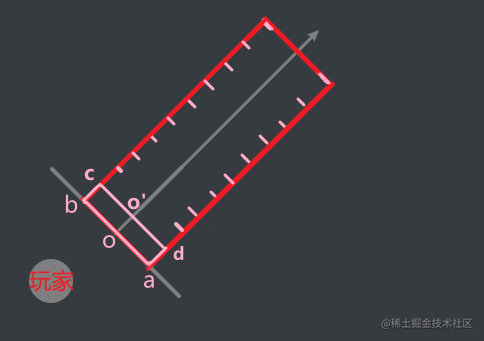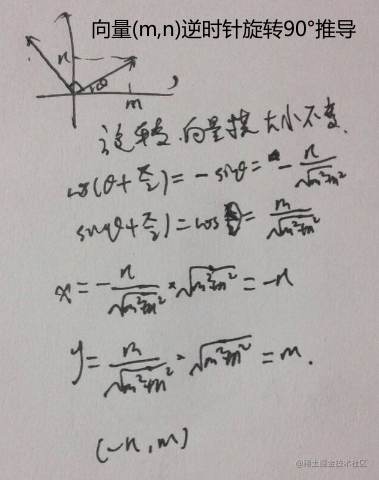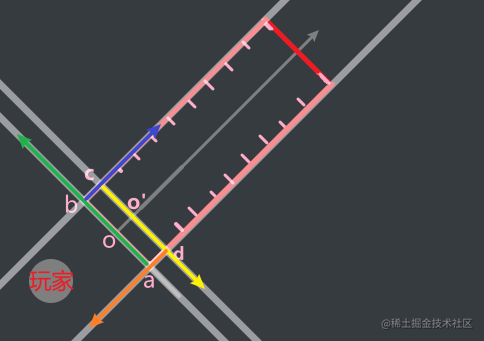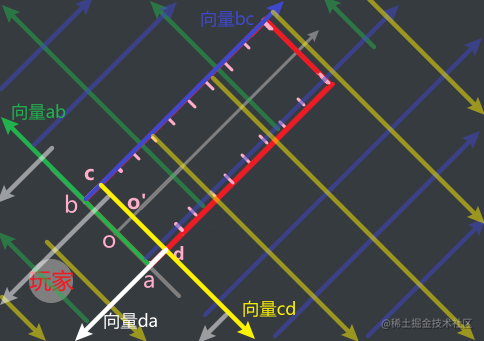# 过程"合理"，表示的是让玩家看到的现象是合理的：

• 剑气的话尽量窄，不要超过剑气特效的宽度
• 假设不是剑气移动而比如说范围内有落石一步一步从近端砸到远端，那就是落石的宽度

(为方便举例，总长为步长的整数倍。如果不是整数倍，那么最后一段的步长=移动终点 - 倒数第二次基 点位置)

o: (p,q) o': (p',q')=o+$\vec{oo'}$*(oo')

$\vec{oa}$ = 顺时针旋转$\vec{oo'}$ = (n,-m)

$\vec{ob}$ = 逆时针旋转$\vec{oo'}$ = (-n,m)

a = o+$\vec{oa}$ * (oa)

b = o+$\vec{ob}$ * (ob)

d = o'+$\vec{oa}$ * (oa)

c = o'+$\vec{oa}$ * (ob)

【附】推导公式# 判断点在矩形内的方法